# Use 3 steps of the Runge-Kutta (fourth order) method to solve the following diﬀerential equation to...

Use 3 steps of the Runge-Kutta (fourth order) method to solve the following diﬀerential equation to t = 2.4, given that y(0) = 2.3. In your working section, you must provide full working for the ﬁrst step. To make calculations easier, round the tabulated value of y at each step to four decimal places.
a) Provide the four K-values that are calculated at the ﬁrst step, with four decimal places. b) Provide your answer for y(2.4) with four decimal places.
(dy /dt) = 1.1ty

## Solutions

##### Expert Solution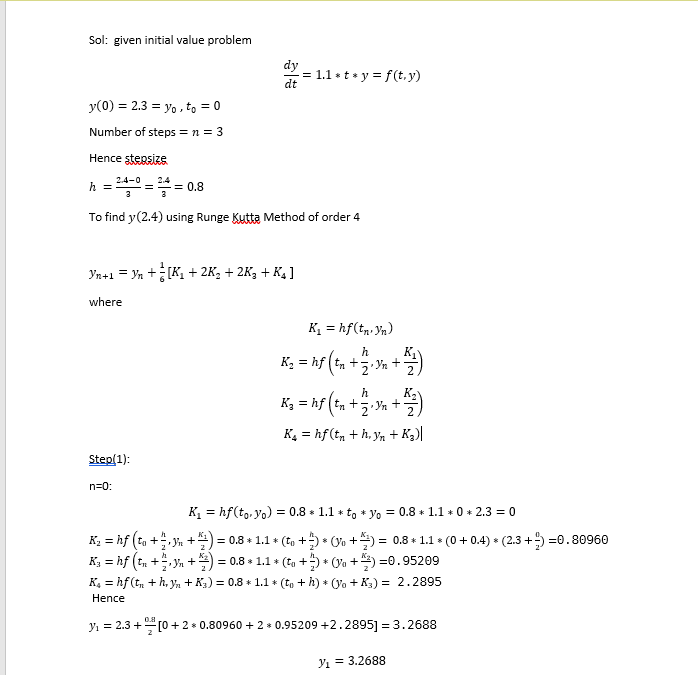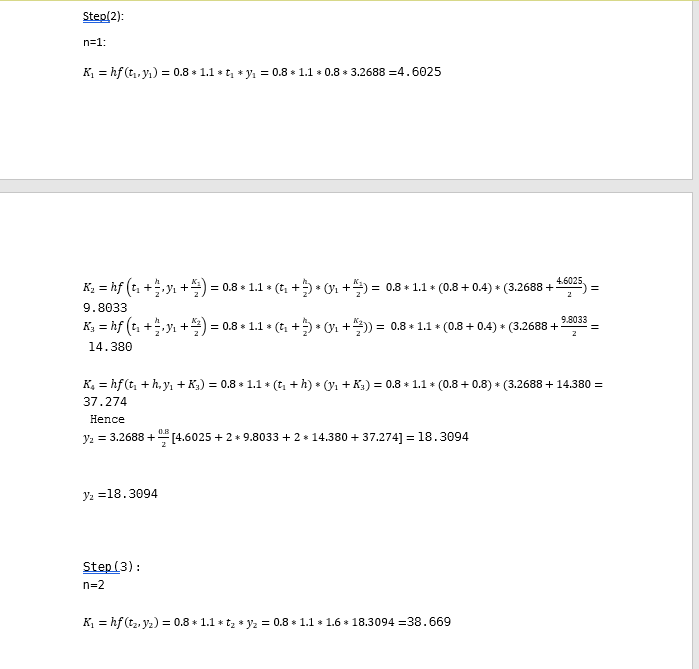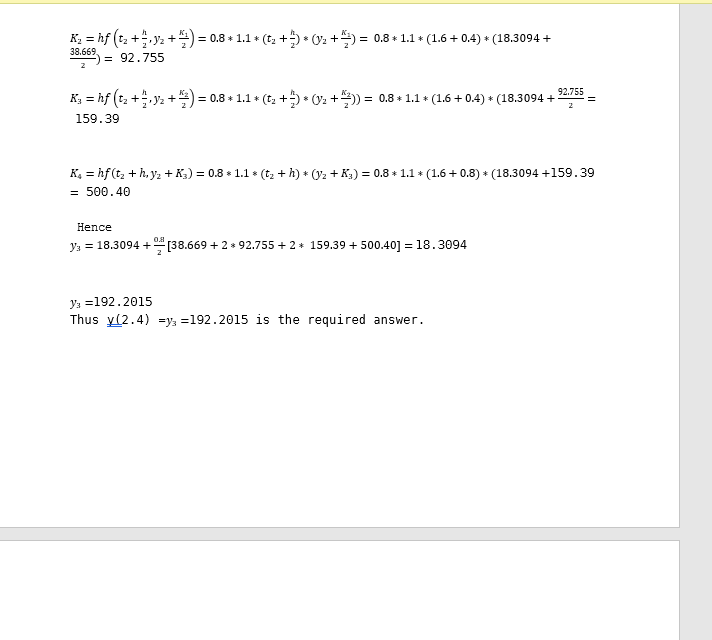##### Expert Solution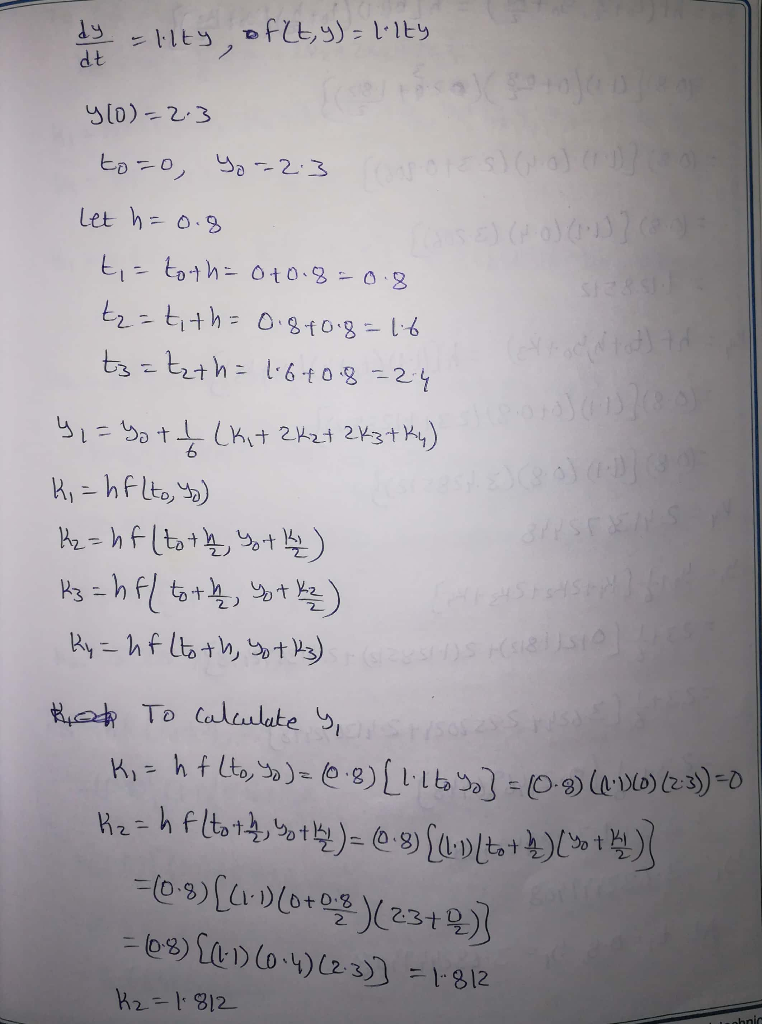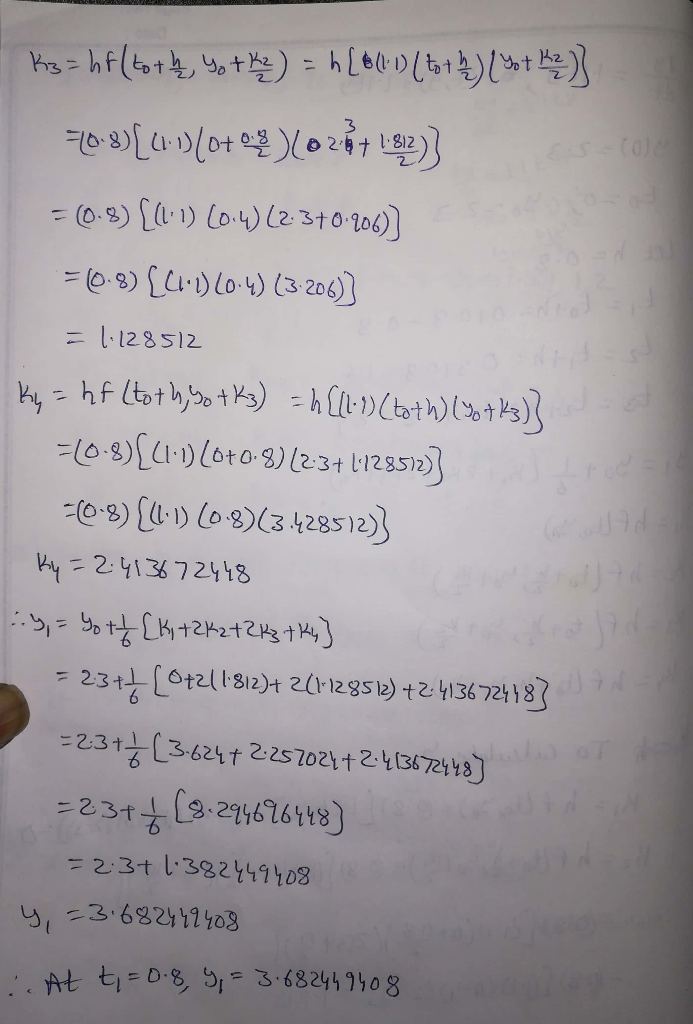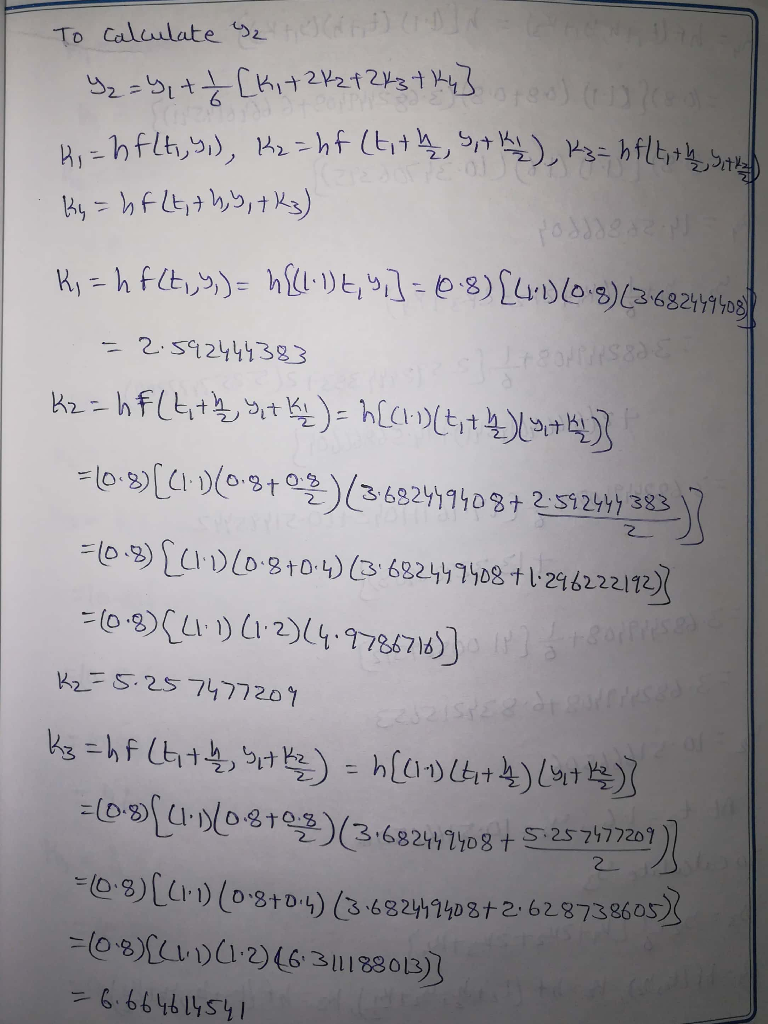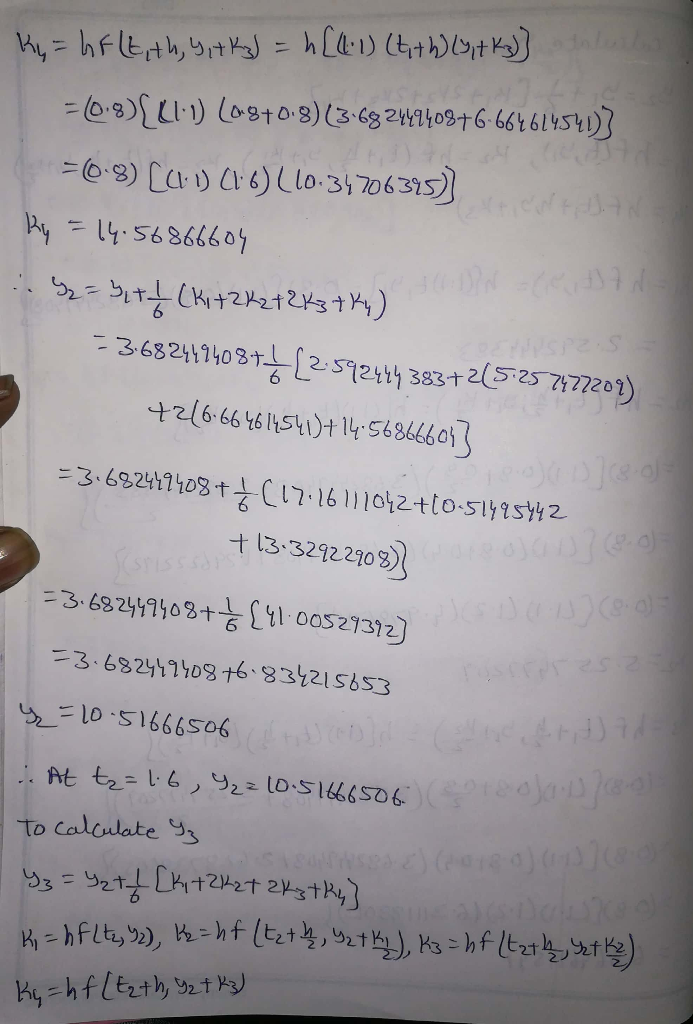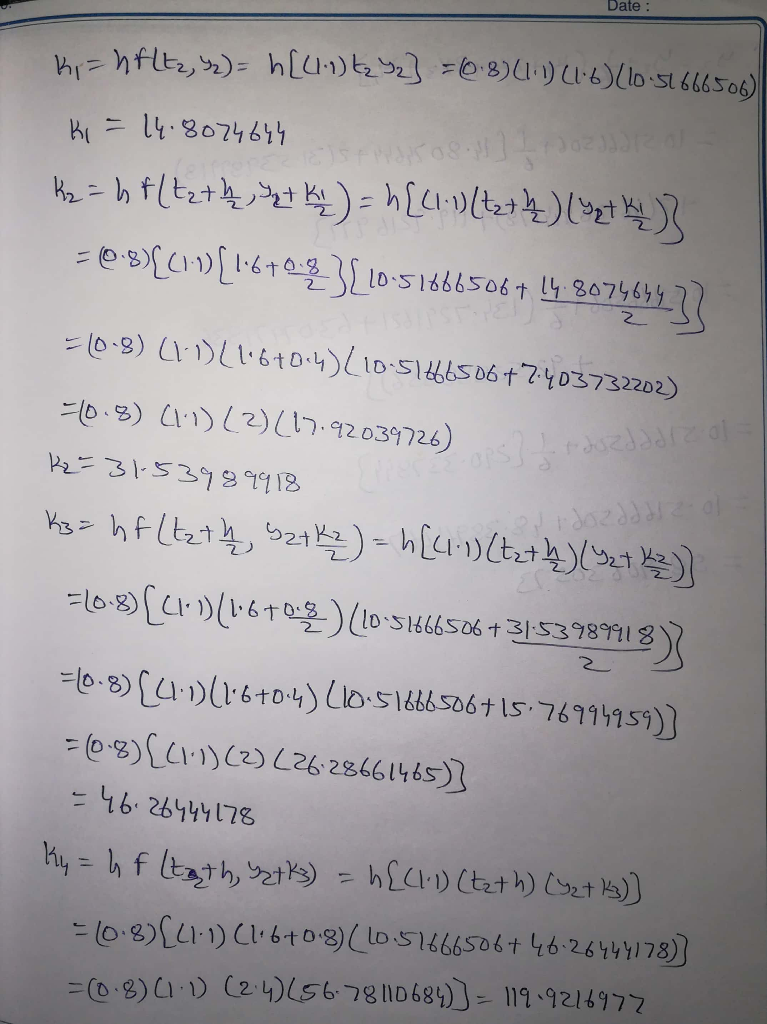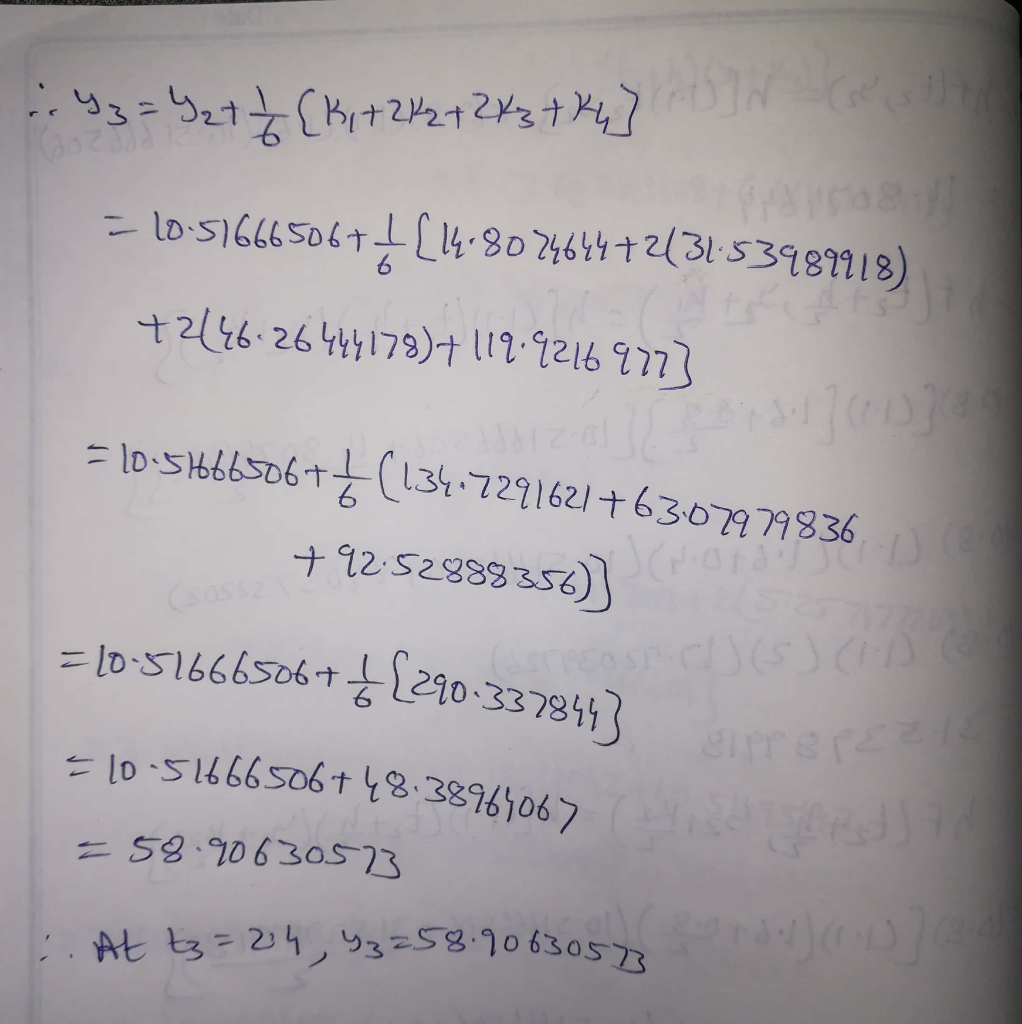## Related Solutions

##### Q 4. With the aid of fourth order Runge-Kutta method, solve the competing species model [20...
Q 4. With the aid of fourth order Runge-Kutta method, solve the competing species model [20 points] defined by dx =x(2 − 0.4x − 0.3y), x(0) = 4 dt dy =y(1 − 0.1y − 0.3x), y(0) = 3 dt where the populations x(t) and y(t) are measured in thousands and t in years. Use a step size of 0.2 for 0 ≤ t ≤ 2 and plot the trajectories of the populations with Matlab or GNU Octave.
##### With the aid of fourth order Runge-Kutta method, solve the competing species model defined by dx/dt...
With the aid of fourth order Runge-Kutta method, solve the competing species model defined by dx/dt =x(2 − 0.4x − 0.3y), x(0) = 2 dy/dt =y(1 − 0.1y − 0.3x), y(0) = 4 where the populations x(t) and y(t) are measured in thousands and t in years. Use a step size of 0.2 for 0 ≤ t ≤ 2 and plot the trajectories of the populations with Matlab or GNU Octave.
##### Write a user-defined MATLAB function that uses classical fourth order Runge-Kutta method to solve a first...
Write a user-defined MATLAB function that uses classical fourth order Runge-Kutta method to solve a first order ODE problem dydx = f(x, y) in a given interval a ? x ? b with initial condition y(a) = y0 and step size of h. For function name and arguments, use [x,y] = myrk4(f, a, b, h, y0) Check your function to find the numerical solution for dydx=?1.2y+7e^(?0.3x) in the interval 0 ? x ? 4 with initial condition y(0)=3. Run your...
##### Use 4 steps of the Modiﬁed Euler’s method to solve the following diﬀerential equation to t...
Use 4 steps of the Modiﬁed Euler’s method to solve the following diﬀerential equation to t = 2.6, given that y(0) = 1.1. In your working section, you must provide full working for the ﬁrst two steps. To make calculations easier, round the calculations at each step to four decimal places, and provide your ﬁnal answer with four decimal places. dy/ dt = 1.4sin(ty)
##### Use Classic Runge-Kutta method with h = 1 to solve the system y” - y’ -...
Use Classic Runge-Kutta method with h = 1 to solve the system y” - y’ - 6y = 0, y(0) = 2, y’(0) = 3 on [0,1]
##### Problem Four (12 Marks) Use Runge Kutta method of order four to approximate the solution of...
Problem Four Use Runge Kutta method of order four to approximate the solution of the initial value problem ?′ + 2? = ??3?, 0 ≤ ? ≤ 1, ?(0) = 0, ???ℎ ℎ = 0.5 Hint: Compute ?(0.5) ??? ?(1)
##### 1)Select all that applies to the Fourth-order Runge-Kutta (RK4) method K subscript 1 equals f left...
1)Select all that applies to the Fourth-order Runge-Kutta (RK4) method K subscript 1 equals f left parenthesis t subscript k comma y subscript k right parenthesis K subscript 2 equals f left parenthesis t subscript k plus h over 2 comma space y subscript k plus h over 2 space K subscript 1 right parenthesis K subscript 3 equals f left parenthesis t subscript k plus h over 2 comma space y subscript k plus h over 2 space K...
##### Use the Runge-Kutta method with step sizes h = 0.1, to find approximate values of the...
Use the Runge-Kutta method with step sizes h = 0.1, to find approximate values of the solution of y' + (1/x)y = (7/x^2) + 3 , y(1) = 3/2 at x = 0.5 . And compare it to thee approximate value of y = (7lnx)/x + 3x/2
##### use Runge Kutta 4th order method y'=y-1.3333*exp(0.6x) a) h=2.5 and compare the value to the exact...
use Runge Kutta 4th order method y'=y-1.3333*exp(0.6x) a) h=2.5 and compare the value to the exact value b) h=1.25 and compare the value to the exact value Thks!
##### Using Runge-Kutta method of order 4 to approximate y(1) with step size h = 0.1 and...
Using Runge-Kutta method of order 4 to approximate y(1) with step size h = 0.1 and h = 0.2 respectively (keep 8 decimals): dy/dx = x + arctan y, y(0) = 0. Solutions: when h = 0.1, y(1) = 0.70398191. when h = 0.2, y(1) = 0.70394257.